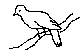# Yet Another TeX mode for Emacs

YaTeX is an intelligent, acquisitive and integrated package which reduces your efforts of composing LaTeX source on Emacs.

YaTeX has its brothers on other distinguished Editors on DOS and Windows, Vz, Wz, Hidemaru, xyzzy. You can write your document with the same interface on any platform if you are using YaTeX family.

And yahtml is the honest and bright YaTeX-compatible major-mode package for writing HTML. If you have noticed the power of YaTeX, you can drive yahtml over the HTML files quickly and steadily. And vice versa, of course.

YaTeX($BLnD;(B)$B$O!"(BEmacs $B$G(B LaTeX $B%=!<%9$r=q$/$H$-$K@8$:$k yahtml$B$OLnD;$N%f!<%6%$%s%?%U%'!<%9$r0z$-7Q$$@(BHTMLBMQN%a%8%c!<(B B%b!<%I%Q%C%1!<%8G9!#(BLaTeX B%=!<%9NF~NOKLnD;,7g+;J$$$H5$$E$$$?$"$J$?$O!"$*$=$i$/(B yahtml $B$K$9$0$J$8$_F1$8$h$&$K;H$$3J;k3HG7g&!#(B BbAms=N5Ub!#(B ## YaTeX (for GNU Emacs/Mule/XEmacs) • Distributions  Release Current 2022/12/25 yatex 1.83 (YaTeX&yahtml) Sign MD5 (yatex1.83.tar.gz) = 9fa32b3e47689044f3196aaaf7d586e6 yatex-hg (Mercurial repo.) yatex-git (GitBucket repo.) yatex-current (test version) rsync (periodical snapshot) • Packaging system specific Instalattion  Linux with apt-get apt-get install yatex FreeBSD pkg install yatex Cask recipe (depends-on "yatex" :hg "http://www.yatex.org/hgrepos/yatex") El-Get recipe (el-get-bundle yatex :type hg :url "http://www.yatex.org/hgrepos/yatex" :branch "dev") From tar-gz archive gzip -dc yatex-VERSION.tar.gz cd yatex-VERSION make install # or "sudo make install" make show-init # Take a look at output make show-init >> ~/.emacs • Manual  Japanese English tex2htmlBKhk(BInfo (BJ?LLE*(B) Info with texi2html(flat) info2wwwBKhk(BInfo (BLZ9=BE*(B) Info with info2www (tree) yahtmlBN(B Info(info2www) yahtml-Info(info2www) PDFBHG(B manual(PDF) Q&A - YaTeX / yahtml Q&A - YaTeX / yahtml B%/%%C%/%j%U%!%l%s%9(B Quick Reference • Links ## LaiTeX (for Vz Editor) - BMkD;(B ## HackTeX (for Wz Editor) - BGrD;(B ## HiTeX (for Hidemaru Editor) - BHtD;(B(BRAg&(B) ## KaTeX (for xyzzy Editor) - B2VD;(B(B+Ag&(B) ## pHackTeX (for Wz Editor ver.4) - pBGrD;(B(BQ/Ag&(B) ## CaTeX (for VSCode) - B7ZD;(B/B2xD;(B(B1Ag&(B) ## The Wild Birds of a feather'' Mailing List BLnD;%a%%j%s%0%j%9%H!V(Bfj BLnD;N2q!WN40FFb(B There is the mailing list for YaTeX (Wild Bird), it's implementation on Vz-Editor, Raicho (LaiTeX; by the late KATSURAGAWA, Naoki , and it's implementation on Wz-Editor, Hackucho (HackTeX; by TAKENAKA, Hiroshi, and it's implementation on Hidemaru-editor, Hichou (HiTeX; by YASUDA, Haruyuki). and it's implementation on xyzzy, Kacho (KaTeX; by Maeda Manabu). Send a mail like  To: yatex@yatex.org Subject: subscribe ----- Hi! I'm yuuji! See any birds fly by?  and you're in. The text will be used as your profile. (you can change it later) Once you're in, you'll get a reply with lists of commands used in the mailing list. Join along and help adding new feathers to Wild Bird. BTW, if you haven't guessed it yet, yes, this mailing list is in Japanese. But give us a try anyway. You're always welcome. BLnD;!"*hS=N(BVzBXN0\?"HGG"kMkD;!"(BWzBXN0\?"HGG"kGrD;!"(BHidemaru BXN0\?"HGG"kHtD;!"(BxyzzyBXN0\?"HGG"k2VD;!"K4X9k(B B>pJs8r49r9k?aN%a%%j%s%0%j%9%H!V(Bfj BLnD;N2q!W,B8:_7^9!#(Byatex@yatex.org BK(B subscribe BH$$$&(B *$B%5%V%8%'%/%H(B* $B$H<+8J>R2p$NJ8>O$rIU$1$?%a!<%k$r=P$9$H!"(B $B<+F0E*$K%a%s%P!<$H$7$FEPO?$5$l$^$9!#$J$*!"<+8J>R2p$NJ8$,L5$$>l9g(B B0l8+2CF~7?h&K8+(^9,!"<+F0E*KEPO?2r=|7^9NG8fCm0U2<5$$!#(B

$B!Z%j%s%/$K$D$$F![(B B3N%Z!<%8XN%j%s%/O8f<+M3KL5CGG*D%j/@5$$!#(B $B%j%s%/$rD%$k>l9g$K(B$B$3$N%^%9%3%C%H(B(80x50gif)$B$b$h$m$7$1$l$P$4MxMQ$/$@$5!#(B

50609
yuuji@yatex.org
HIROSE Yuuji - ASTROLOGY / BIKE / EPO / GUEST BOOK / YaTeX